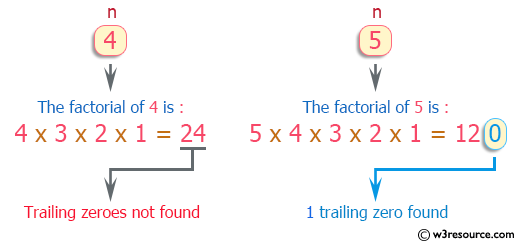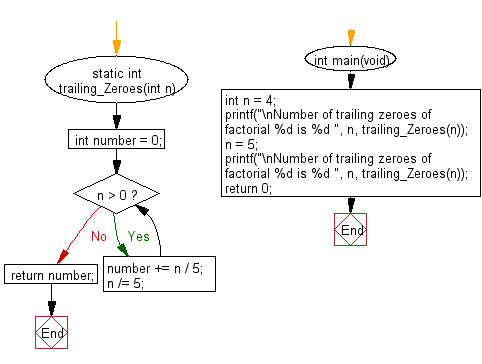﻿ C Program: Number of trailing zeroes in a factorial

# C Exercises: Find the number of trailing zeroes in a given factorial

## C Programming Mathematics: Exercise-10 with Solution

Write a C program to find the number of trailing zeroes in a given factorial.

Example 1:
Input: 4
Output: 0
Explanation: 4! = 24, no trailing zero.
Example 2:
Input: 6
Output: 1
Explanation: 6! = 720, one trailing zero.

Example:
Input:
n = 4
n = 5
Output:
Number of trailing zeroes of factorial 4 is 0
Number of trailing zeroes of factorial 5 is 1

Pictorial Presentation:Sample Solution:

C Code:

``````#include <stdio.h>

static int trailing_Zeroes(int n)
{
int number = 0;
while (n > 0) {
number += n / 5;
n /= 5;
}
return number;
}
int main(void)
{
int n = 4;
printf("\nNumber of trailing zeroes of factorial %d is %d ", n, trailing_Zeroes(n));
n = 5;
printf("\nNumber of trailing zeroes of factorial %d is %d ", n, trailing_Zeroes(n));
return 0;
}
```
```

Sample Output:

```Number of trailing zeroes of factorial 4 is 0
Number of trailing zeroes of factorial 5 is 1
```

Flowchart:-->

C Programming Code Editor:

Improve this sample solution and post your code through Disqus.

What is the difficulty level of this exercise?

Test your Programming skills with w3resource's quiz.

﻿